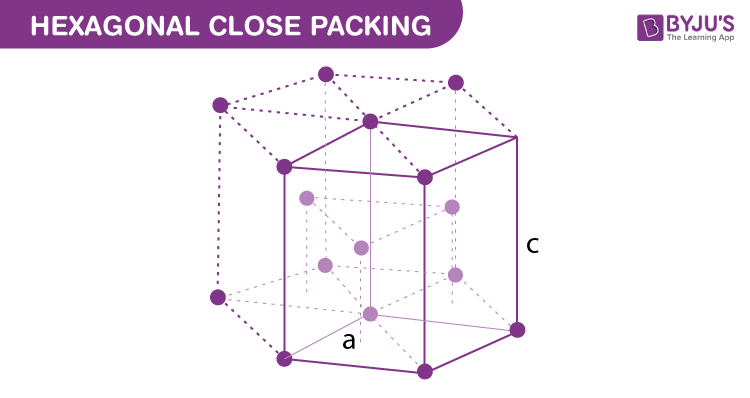Jet Set Go! All about Aeroplanes Jet Set Go! All about Aeroplanes

# Hexagonal Close Packing Formula

Crystalline solids exhibit a regular and repeating pattern of constituent particles. The diagrammatic representation of three-dimensional arrangements of constituent particles in a crystal, in which each particle is depicted as a point in space is known as a crystal lattice. In a crystal lattice, the atoms are very closely packed, leaving very little space between them. This arrangement of elements in solids also helps us in the determination of the formula of a compound. We have learned three-dimensional solid packing can be packed in two ways viz., cubical close packing (CCP) and hexagonal close packing (HCP).

## Hexagonal Close PackingIn hexagonal close packing (HCP) too, there are two basic kinds of voids are involved, namely, octahedral voids and tetrahedral voids. We know that the number of tetrahedral voids present in a lattice is twice the number of close-packed particles. While the number of octahedral voids generated is equal to the number of close-packed particles. The arrangement of particles in these voids depends on other factors too. For example, in ionic solids, the bigger ions from the close-packed structure and the smaller ions occupy the voids. Tetrahedral voids are occupied if the latter ions are small. Whereas if the latter ions are bigger, octahedral voids are occupied. The fraction of octahedral or tetrahedral voids occupied by the molecules helps us in the determination of the formula of the compound.

### Problems on hexagonal close packing Formula

Question: Atoms of element Y form hexagonal close packing lattice and those of the element X occupy 1/4th of tetrahedral voids. What is the formula of the compound formed by the elements X and Y?

Solution: The number of tetrahedral voids formed = 2 × (number of atoms of element Y)

Since only 1/4th of these voids are occupied by X, the ratio of elements of X to Y can be given by:

2 × (1/4):1 or 1:2

Thus, the formula of the compound is XY2.

## Frequently Asked Questions – FAQs

### What is close packing in solids?

Near crystal, packing refers to the effective arrangement of constituent particles in a crystal lattice in a vacuum. We have to assume that all particles (atoms, molecules and ions) are of the same spherical solid form to grasp this set more precisely. So a cubic structure is the unit cell of a lattice.

### How many types of close packing are known in crystals?

Inside a unit cell, the most powerful conformation atomic spheres can take is known as the nearest packing configuration. In two modes, there are closely packed atomic spheres: hexagonal closest packing (HCP) and cubic closest packing (CCP).

### What is the difference between hexagonal close packing and cubic close packing?

The tight packing of the hexagon is denoted as HCP. In one repeated unit, this arrangement has two layers of spheres. The main distinction between hexagonal close packing and cubic close packing is that there are 6 spheres in a unit cell of hexagonal close packing, whereas there are 4 spheres in a unit cell of cubic close packing.

### What is hexagonal packing?

Hexagonal tightly packed (hcp) refers to layers of packed spheres such that spheres overlay each other in alternating layers. A slip framework, which is a close-packed structure, is hexagonal near packed. For elemental metals, the hcp structure is very general, including beryllium.

For a detailed discussion on hexagonal close packing and cubical close packing, download BYJU’S – The Learning App.

Take up a quiz on Hexagonal Close Packing Formula